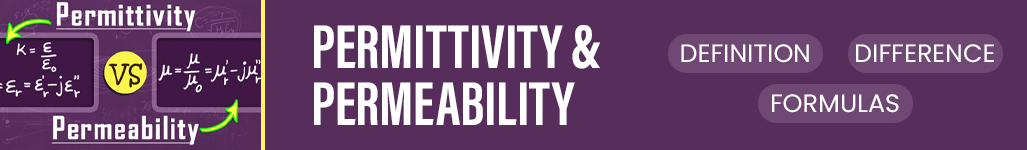Permittivity and Permeability: Definition, Difference & Form - Study24x7New to Study24X7 ?

# Permittivity and Permeability: Definition, Difference & Formulas

Updated on 21 January 2021Class12NonMedical
Updated on 21 January 2021Permittivity and permeability are the most important concepts of electromagnetic theory. The fundamental definitions of permittivity and permeability are as follows- Permittivity measures the hindrance caused by the material while the electrical field is formed. In simpler terms Permittivity is an electrical field concept.

Whereas permeability computes the effectiveness of the material which allows the magnetic lines of force to pass through it. Thus it states that Permeability is a magnetic field concept.

In this article, we will see the permittivity and permeability relation to better understand these topics in detail.

## What is Permittivity?

The concept related to the generation of the electrical field is known as permittivity. They can be defined as the ratio of electric displacement to the electric field intensity. It represents the quality of a material that causes opposition during electric current development.

The permittivity of a material is denoted by symbol ϵ whereas the SI unit is farad per meter and has the value of 8.85x10-12 Farad/meter.

Permittivity measures the total number of changes needed for generating a unit of electric current in a specified medium.

## What is Permeability?

Permeability is the property of the material that supports the emergence of a magnetic field. Permeability is affected by many factors such as temperature, field strength, frequency, and humidity level.

It is denoted by μ. However, the permeability of free space, commonly known as constant permeability and is denoted by μ0, which approximately equals to 4Π X 10-7Henry/meter.## Key Difference between Permittivity and Permeability

 Basis of Comparison Permittivity Permeability Meaning Measure the hindrance caused by the material in the formation of electrical influx. Measure the property of the material that supports the advent of the magnetic field Denoted by ϵ μ SI Unit Farad/Meter Henry/Meter Formula Electric displacement/ Electric field intensity The magnitude of magnetic induction/ Intensity of magnetic field Field Electric field Magnetic field Free Space Value Free space of permittivity= 8.85x10-12Farad/meter Free space of permeability= 4𝝅x 10-7

## Relative Permittivity

Relative permittivity is also known as the dielectric constant is the factor by which charges between the electrical fields decrease relative to vacuum. In simpler words, relative permittivity is the ratio of the permittivity of the substance to the permittivity of the space or the vacuum.

Relative permittivity is typically denoted as εr(ω) which is proportionally constant between electric field and electric displacement.

## Permeability of Free Space

Permeability of free space is also known as permeability constant which represents the capability of Vacuum to permit electrical fields. it is denoted by μ0= 4𝝅×10-7 H/m. This is a scalar quantity for an isotropic medium.

## Conclusion

Above we have discussed the Permittivity and permeability relation. These both topics are the most common as well as important concepts of physics. Permittivity states the challenges to form an electrical field within a medium. In the same manner, permeability measures the ability of the material to form an electrical field. The major difference between the two is permeability does not mention the difficulty or easy level however permittivity does.

Related Article:

Nuclear fusion and fission

Write a comment...Trending ArticlesBy CBSE CLASS 8By CBSE CLASS 10By Examination GuideBy CBSE CLASS 9